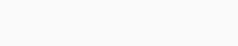# Python | Understanding Style Transfer using CNNs

Hello folks! In this article, we are going to see how we can transfer the style of one image to another image. Among the applications of convolutional neural networks (CNN) and visual recognition, style transfer has been a very heated topic. Style transfer is the technique of separating and recombining the content and the style of an arbitrary image. So before going to the main topic let us discuss terminologies.

## What is Style Transfer?

Style transfer is the technique of separating and recombining the content and the style of an arbitrary image. It is a technique that combines both artistic aspects and recognition (content) aspects of images.

## Procedure for obtaining Styled image.-

Here we will use two images, one content image and other style image and will get one output image. Once we determine which layers are used to represent content and style we will use the content loss by calculating the difference at layer between input image and output image.Style loss by calculating difference at layer between style image and output image and Variation loss by calculating difference at layer between input and output images. Since the response layers of images of different sizes cannot be compared directly we use the Gram matrix of representations for style loss calculation.Once we obtain all the losses we will minimize total loss. Style transfer problem is now a mathematical problem!

In the end, we set our hyper-parameters and optimize the results with L-BFGS algorithm.

## Code: Style Transfer using CNNs in Python

```#Input visualization
input_image = Image.open(BytesIO(requests.get(image_path).content))
input_image = input_image.resize((IMAGE_WIDTH, IMAGE_HEIGHT))
input_image.save(input_image_path)
input_image

#Style visualization
style_image = Image.open(BytesIO(requests.get(image_path).content))
style_image = style_image.resize((IMAGE_WIDTH, IMAGE_HEIGHT))
style_image.save(style_image_path)
style_image

#Data normalization and reshaping from RGB to BGR
input_image_array = np.asarray(input_image, dtype="float32")
input_image_array = np.expand_dims(input_image_array, axis=0)
input_image_array[:, :, :, 0] -= IMAGENET_MEAN_RGB_VALUES
input_image_array[:, :, :, 1] -= IMAGENET_MEAN_RGB_VALUES
input_image_array[:, :, :, 2] -= IMAGENET_MEAN_RGB_VALUES
input_image_array = input_image_array[:, :, :, ::-1]

style_image_array = np.asarray(style_image, dtype="float32")
style_image_array = np.expand_dims(style_image_array, axis=0)
style_image_array[:, :, :, 0] -= IMAGENET_MEAN_RGB_VALUES
style_image_array[:, :, :, 1] -= IMAGENET_MEAN_RGB_VALUES
style_image_array[:, :, :, 2] -= IMAGENET_MEAN_RGB_VALUES
style_image_array = style_image_array[:, :, :, ::-1]

#Model
input_image = backend.variable(input_image_array)
style_image = backend.variable(style_image_array)
combination_image = backend.placeholder((1, IMAGE_HEIGHT, IMAGE_SIZE, 3))

input_tensor = backend.concatenate([input_image,style_image,combination_image], axis=0)
model = VGG16(input_tensor=input_tensor, include_top=False)

#Content_Loss
def content_loss(content, combination):
return backend.sum(backend.square(combination - content))

layers = dict([(layer.name, layer.output) for layer in model.layers])

content_layer = "block2_conv2"
layer_features = layers[content_layer]
content_image_features = layer_features[0, :, :, :]
combination_features = layer_features[2, :, :, :]

loss = backend.variable(0.)
loss += CONTENT_WEIGHT * content_loss(content_image_features,
combination_features)

# Style_loss
def gram_matrix(x):
features = backend.batch_flatten(backend.permute_dimensions(x, (2, 0, 1)))
gram = backend.dot(features, backend.transpose(features))
return gram

def compute_style_loss(style, combination):
style = gram_matrix(style)
combination = gram_matrix(combination)
size = IMAGE_HEIGHT * IMAGE_WIDTH
return backend.sum(backend.square(style - combination)) / (4. * (CHANNELS ** 2) * (size ** 2))

style_layers = ["block1_conv2", "block2_conv2", "block3_conv3", "block4_conv3", "block5_conv3"]
for layer_name in style_layers:
layer_features = layers[layer_name]
style_features = layer_features[1, :, :, :]
combination_features = layer_features[2, :, :, :]
style_loss = compute_style_loss(style_features, combination_features)
loss += (STYLE_WEIGHT / len(style_layers)) * style_loss

# Total variation loss
def total_variation_loss(x):
a = backend.square(x[:, :IMAGE_HEIGHT-1, :IMAGE_WIDTH-1, :] - x[:, 1:, :IMAGE_WIDTH-1, :])
b = backend.square(x[:, :IMAGE_HEIGHT-1, :IMAGE_WIDTH-1, :] - x[:, :IMAGE_HEIGHT-1, 1:, :])
return backend.sum(backend.pow(a + b, TOTAL_VARIATION_LOSS_FACTOR))

loss += TOTAL_VARIATION_WEIGHT * total_variation_loss(combination_image)

outputs = [loss]
outputs += backend.gradients(loss, combination_image)

x = x.reshape((1, IMAGE_HEIGHT, IMAGE_WIDTH, CHANNELS))
outs = backend.function([combination_image], outputs)([x])
loss = outs

class Evaluator:

def loss(self, x):
return loss

evaluator = Evaluator()

#LFGS_Algorithm
x = np.random.uniform(0, 255, (1, IMAGE_HEIGHT, IMAGE_WIDTH, 3)) - 128.

for i in range(ITERATIONS):
x, loss, info = fmin_l_bfgs_b(evaluator.loss, x.flatten(), fprime=evaluator.gradients, maxfun=20)
print("Iteration %d completed with loss %d" % (i, loss))

x = x.reshape((IMAGE_HEIGHT, IMAGE_WIDTH, CHANNELS))
x = x[:, :, ::-1]
x[:, :, 0] += IMAGENET_MEAN_RGB_VALUES
x[:, :, 1] += IMAGENET_MEAN_RGB_VALUES
x[:, :, 2] += IMAGENET_MEAN_RGB_VALUES
x = np.clip(x, 0, 255).astype("uint8")
output_image = Image.fromarray(x)
output_image.save(output_image_path)
output_image```

Style Image:-Output Image:-This is the code you can read comments out there for better understanding. We used the same procedure as stated above. Thank you!